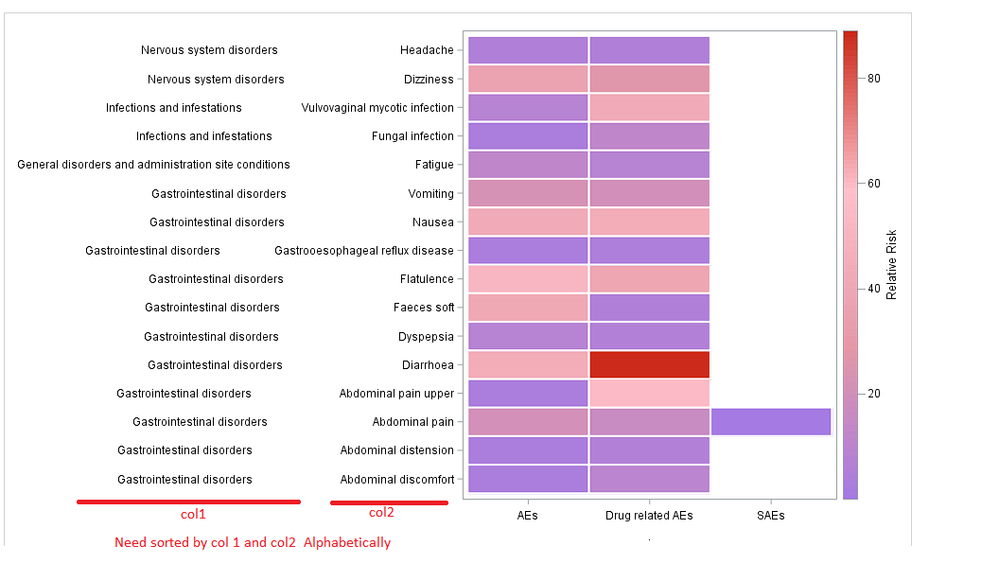## How to sort alphabetically for heatmap

Hi,

I want the display the data sorted alphabetically in column 1 and 2.

However, it is being sorted in descending order as shown in screenshot.

How to sort it alphabetically ?

In general, how the sort order will be controlled ?

``````DATA WORK.Book1;
infile datalines delimiter='^';
LENGTH
AEBODSYS         \$ 52
AEDECOD          \$ 32
cnt_ae             8
cnt_aerel          8
cnt_sae            8 ;
FORMAT
AEBODSYS         \$CHAR52.
AEDECOD          \$CHAR32.
cnt_ae           BEST12.
cnt_aerel        BEST12.
cnt_sae          BEST12. ;
INFORMAT
AEBODSYS         \$CHAR52.
AEDECOD          \$CHAR32.
cnt_ae           BEST12.
cnt_aerel        BEST12.
cnt_sae          BEST12. ;
INFILE DATALINES4
/*        DLM='7F'x*/
MISSOVER
DSD ;
INPUT
AEBODSYS         : \$CHAR52.
AEDECOD          : \$CHAR32.
cnt_ae           : BEST32.
cnt_aerel        : BEST32.
cnt_sae          : BEST32. ;
DATALINES4;
Gastrointestinal disorders^Diarrhoea^45^89^.
Gastrointestinal disorders^Nausea^44^45^.
Gastrointestinal disorders^Flatulence^52^40^.
Gastrointestinal disorders^Faeces soft^42^6^.
Nervous system disorders^Dizziness^38^28^.
Gastrointestinal disorders^Vomiting^23^21^.
Gastrointestinal disorders^Abdominal pain^22^17^1
Gastrointestinal disorders^Abdominal pain upper^4^55^.
Infections and infestations^Fungal infection^4^13^.
Gastrointestinal disorders^Abdominal discomfort^4^11^.
General disorders and administration site conditions^Fatigue^13^9^.
Infections and infestations^Vulvovaginal mycotic infection^9^44^.
Gastrointestinal disorders^Abdominal distension^4^7^.
Gastrointestinal disorders^Dyspepsia^9^7^.
Gastrointestinal disorders^Gastrooesophageal reflux disease^4^5^.
;;;;
run;

data  b2a ;
length cat \$20 yax \$300 ;
set
Book1 (where= (cnt_ae ne . ) in=a )
Book1 (where= (cnt_aerel ne . ) in=b)
Book1 (where= (cnt_sae ne . )in=c)
;
if a then do ; cat='AEs'; var= cnt_ae ; end;
if b then do ; cat='Drug related AEs'; var= cnt_aerel ; end;
if c then do ; cat='SAEs'; var= cnt_sae ; end;

run;

proc sort data= b2a; by  cat  aebodsys aedecod; run;

data  b2    ;
length   yax \$300 ;
set  b2a ;
/*by descending cat descending aebodsys;*/
/*lag=lag(aebodsys);*/
/*if aebodsys=lag then aebodsys=''; */
length aebodsys2 \$80 aedecod2 \$50;
aebodsys2=aebodsys ;
aedecod2=aedecod
;
/* yax=put (aebodsys2 ,\$80. -r) || '               '|| right ( put (aedecod ,\$50. -r) ); */
yax=right (aebodsys2 ) || ' '|| right (aedecod2 );

run;

proc template;
define statgraph heatmap;
begingraph / designwidth=700 designheight=500;
entrytitle '';
rangeattrmap name="rmap";
/*	    range 0 - max / rangecolormodel=(VLIPB LIPK VIYPK );*/
range 0 - max / rangecolormodel=(VLIPB LIPK pink VIYPK );
endrangeattrmap;
rangeattrvar attrmap="rmap" var= var /*  cnt_aerel cnt_sae */ attrvar=pColor;
layout overlay / xaxisopts =(label='.') yaxisopts=(display=(ticks tickvalues line) )  ;
heatmapparm x=cat  /* cnt_aerel cnt_aerel cnt_sae */ y=yax  /* aedecod */ colorresponse=pColor /
xboundaries=(10 30 60 90 120 150 180 210 )
xvalues=leftpoints xendlabels=true
display=all
outlineattrs=graphdata2(thickness=2 color=white )
/*						  outlineattrs= color=white*/
name="heatmap";

continuouslegend "heatmap" / title="Relative Risk";
endlayout;
endgraph;
end;
run;

ods html close;
ods listing style=htmlblue image_dpi=150  ;
ods graphics / reset width=10in height=6in imagename='heatmap';

proc sort data=b2 ; by aebodsys  ; run;

proc sgrender data=b2 template=heatmap; run;``````
` `1 ACCEPTED SOLUTION

Accepted Solutions

## Re: How to sort alphabetically for heatmap

@Peter0123 wrote:

Hi,

I want the display the data sorted alphabetically in column 1 and 2.

However, it is being sorted in descending order as shown in screenshot.

Actually it isn't. The "lowest" value goes next to the X axis. Just like with data in an scatter plot. Y=0 will be at the bottom compared to Y=200.

```	  layout overlay / xaxisopts =(label='.') yaxisopts=(display=(ticks tickvalues line) reverse=true)  ;
```

## Re: How to sort alphabetically for heatmap

@Peter0123 wrote:

Hi,

I want the display the data sorted alphabetically in column 1 and 2.

However, it is being sorted in descending order as shown in screenshot.

Actually it isn't. The "lowest" value goes next to the X axis. Just like with data in an scatter plot. Y=0 will be at the bottom compared to Y=200.

```	  layout overlay / xaxisopts =(label='.') yaxisopts=(display=(ticks tickvalues line) reverse=true)  ;